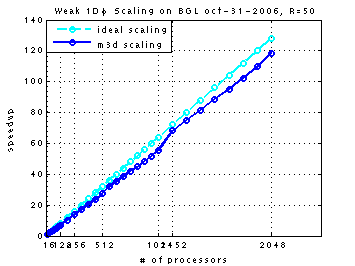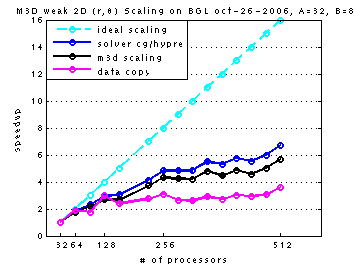document.write(document.title)

We have six parameters (A, B, C, D, E, F) representing the partition of mesh and cpus in M3D calculations.

A gives the total number of planes in toroidal φ direction; B gives the number of CPUs in toroidal φ direction (B ≤ A).
C gives the number of grids in minor radial r direction; D gives the number of CPUs in radial r direction.
E gives the number of partitions in poloidal θ direction (E ≥ 3); F gives the number of CPUs in poloidal θ direction.

The total size of 3D mesh is given by parameters A, C, and E using formulae A[1+C(C-1)E ⁄ 2]; and
the total number of cpu is given by paramters B, D, and F using formulae BF (when D=1) or B(F+1) (when D=2).

Each point in the following plots has individual values assigned to these parameters.
In the case of strong scaling, parameters A, C, and E are fixed all the time, while parameters B, D, and F changes, resulting in an increase of total cpus and the amount of work on each processor is reduced.
In the case of weak scaling, parameters A, C, and E increases as the parameters B, D, and F increases, resulting in a roughly fixed amount of work on each processor all the time.

‡ Seaborg at NERSC: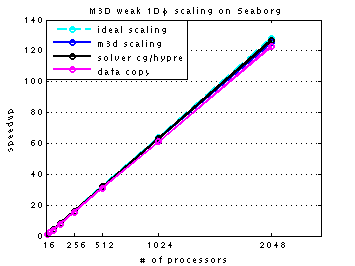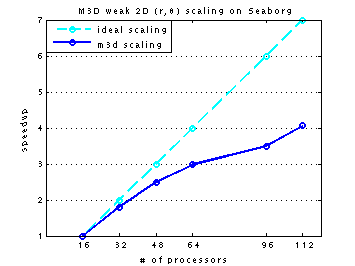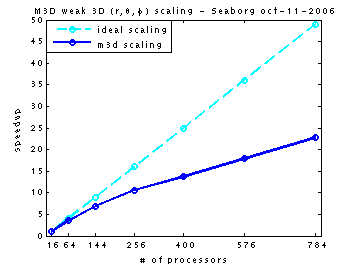3D weak scaling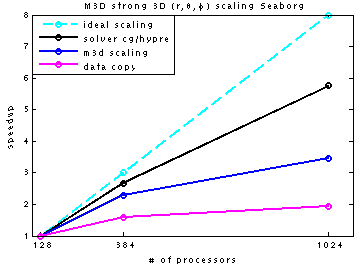‡ Jaguar at ORNL:

1D weak scaling (SN)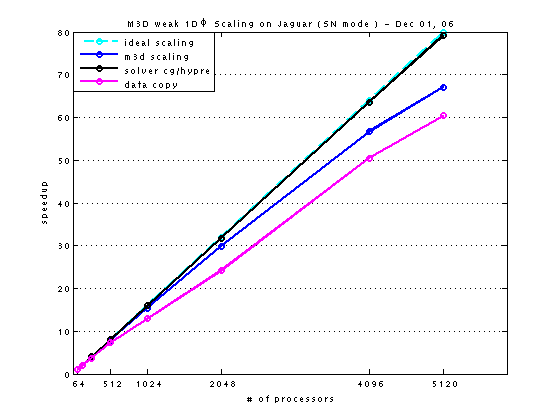1D weak scaling (VN)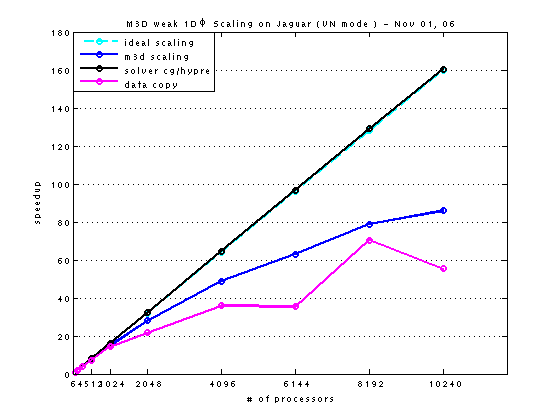Base run: 16 toroidal planes, 560 radial grids, 4 partitions in polodal direction.
average_number_of_vertices_per_cpu_at_point = 39481 is the work size kept for all the eight runs (given as points) in the series of 1D weak scaling.
From point one to eight, the total number of toroidal planes increases 16 in each next run, and the number of cpu in toroidal direction increases 4 so the the work size is kept the same as in the first run.
The eight points in the plot are given by:
(A, B, C, D, E, F)_0064 = (0016, 004, 560, 2, 4, 15)
(A, B, C, D, E, F)_0128 = (0032, 008, 560, 2, 4, 15)
(A, B, C, D, E, F)_0256 = (0064, 016, 560, 2, 4, 15)
(A, B, C, D, E, F)_0512 = (0128, 032, 560, 2, 4, 15)
(A, B, C, D, E, F)_1024 = (0256, 064, 560, 2, 4, 15)
(A, B, C, D, E, F)_2048 = (0512, 128, 560, 2, 4, 15)
(A, B, C, D, E, F)_4096 = (1024, 256, 560, 2, 4, 15)
(A, B, C, D, E, F)_5120 = (1208, 320, 560, 2, 4, 15)

Base run: 16 toroidal planes, 398 radial grids, 4 partitions in polodal direction.
average_number_of_vertices_per_cpu_at_point = 19801 is the work size kept for all the ten runs (given as points) in the series of 1D weak scaling.
From point one to ten, the total number of toroidal planes increases 16 in each next run, and the number of cpu in toroidal direction increases 4 so the the work size is kept the same as in the first run.
The ten points in the plot are given by:
(A, B, C, D, E, F)_00064 = (0016, 004, 398, 2, 4, 15)
(A, B, C, D, E, F)_00128 = (0032, 008, 398, 2, 4, 15)
(A, B, C, D, E, F)_00256 = (0064, 016, 398, 2, 4, 15)
(A, B, C, D, E, F)_00512 = (0128, 032, 398, 2, 4, 15)
(A, B, C, D, E, F)_01024 = (0256, 064, 398, 2, 4, 15)
(A, B, C, D, E, F)_02048 = (0512, 128, 398, 2, 4, 15)
(A, B, C, D, E, F)_04096 = (1024, 256, 398, 2, 4, 15)
(A, B, C, D, E, F)_06144 = (1536, 384, 398, 2, 4, 15)
(A, B, C, D, E, F)_08192 = (2048, 512, 398, 2, 4, 15)
(A, B, C, D, E, F)_10240 = (2560, 640, 398, 2, 4, 15)

3D weak scaling (SN)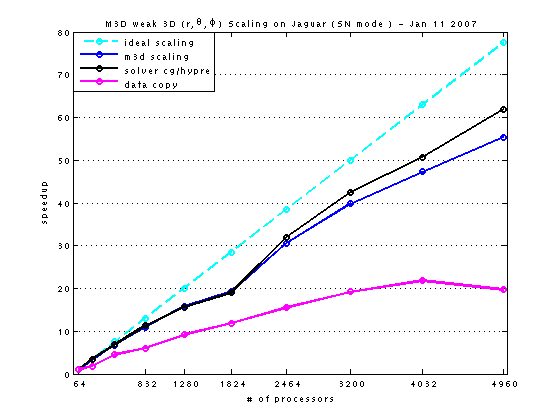Base run: 64 toroidal planes, 283 radial grids, 4 partitions in polodal direction.
average_number_of_vertices_per_cpu_at_point = 39904 is the work size kept for all the ten runs (given as points) in the series of 3D weak scaling.
From point one to ten, the total number of toroidal planes increases 48 in each next run, and the number of cpu in toroidal direction increases 12. The radial grids and poloidal partitions are also increased so the the work size is kept roughly the same as in the first run.
The ten points in the plot are parameterized by:
(A, B, C, D, E, F)_0064 = (064, 016, 283, 1, 04, 04)
(A, B, C, D, E, F)_0224 = (112, 028, 356, 2, 05, 07)
(A, B, C, D, E, F)_0480 = (160, 040, 398, 2, 06, 11)
(A, B, C, D, E, F)_0832 = (208, 052, 427, 2, 07, 15)
(A, B, C, D, E, F)_1280 = (256, 064, 446, 2, 08, 19)
(A, B, C, D, E, F)_1824 = (304, 076, 459, 2, 09, 23)
(A, B, C, D, E, F)_2464 = (352, 088, 469, 2, 10, 27)
(A, B, C, D, E, F)_3200 = (400, 100, 479, 2, 11, 31)
(A, B, C, D, E, F)_4032 = (448, 112, 487, 2, 12, 35)
(A, B, C, D, E, F)_4960 = (496, 124, 491, 2, 13, 39)

3D strong scaling (SN-1)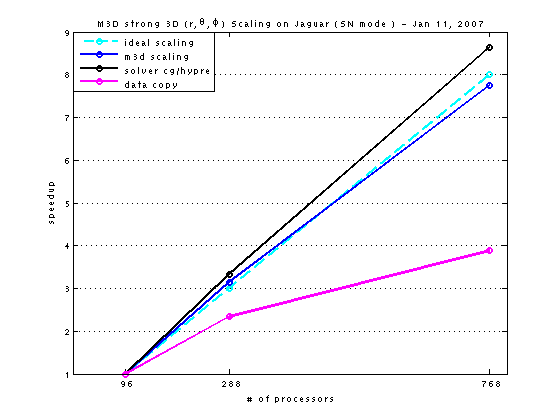3D strong scaling (SN-2)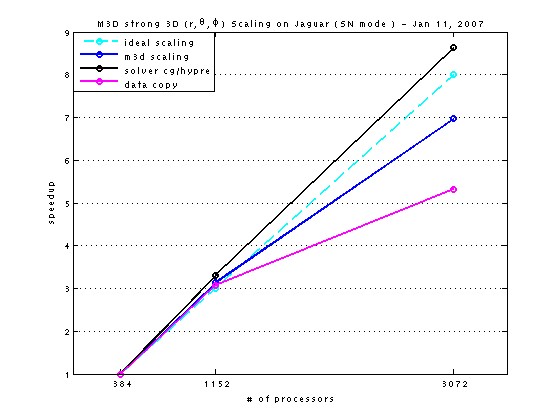3D strong scaling (SN-3)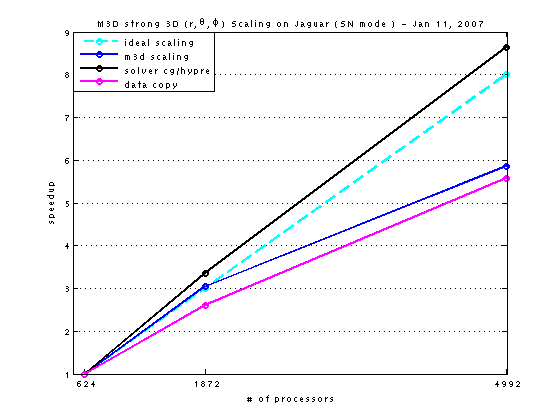Base run: 032 toroidal planes, 436 radial grids, 5 partitions in polodal direction.
The three points in the plot are given by:
(A, B, C, D, E, F)_096 = (32, 08, 436, 2, 5, 11)
(A, B, C, D, E, F)_288 = (32, 16, 436, 2, 5, 17)
(A, B, C, D, E, F)_768 = (32, 16, 436, 2, 5, 23)

Base run: 128 toroidal planes, 436 radial grids, 5 partitions in polodal direction.
The three points in the plot are given by:
(A, B, C, D, E, F)_0384 = (128, 032, 436, 2, 5, 11)
(A, B, C, D, E, F)_1152 = (128, 064, 436, 2, 5, 17)
(A, B, C, D, E, F)_3072 = (128, 128, 436, 2, 5, 23)

Base run: 208 toroidal planes, 436 radial grids, 5 partitions in polodal direction.
The three points in the plot are given by:
(A, B, C, D, E, F)_0624 = (208, 052, 436, 2, 5, 11)
(A, B, C, D, E, F)_1872 = (208, 104, 436, 2, 5, 17)
(A, B, C, D, E, F)_4992 = (208, 208, 436, 2, 5, 23)

Note:
All the above three series of strong scaling runs (SN-1, SN-2, SN_3)
differ only in the total number of toroidal planes:
SN-1 run has 32 planes;
SN-2 run has 128 planes;
SN-3 run has 208 planes.

In all the three runs:
average_number_of_vertices_per_cpu_at_point_1 = 39376;
average_number_of_vertices_per_cpu_at_point_2 = 26266;
average_number_of_vertices_per_cpu_at_point_3 = 20026.

‡ BGL at Argonne: